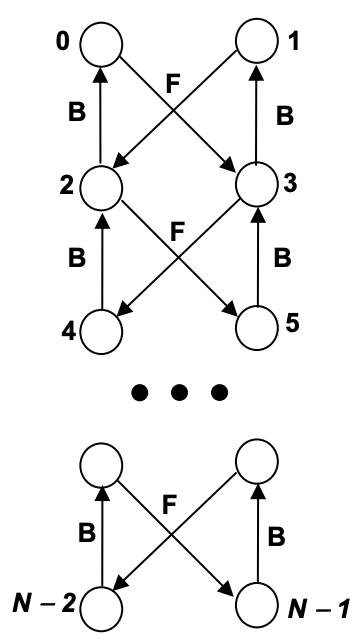시간 제한 메모리 제한 제출 정답 맞은 사람 정답 비율
1 초 128 MB 0 0 0 0.000%

## 문제Billy is a hard-working student. He is fond of computers and intends to learn as much as possible. Now he studies graph theory, and must write a program to build the graph which is shown on the Figure.

The vertices of the graph are labeled sequentially with integer keys starting from 0 to N ≤ 1 (N≤10000). There are two types of edges: backward edges - labeled with B in the Figure (for example from node 4 to node 2, or from node 3 to node 1), and forward edges, labeled with F in the Figure (for example from node 1 to node 2 or from node 0 to node 3). Billy’s program starts with an initial graph that contains the vertices 0, 1, 2, 3, and must continue to build the graph based on a sequence of commands written in a text file. A command has the following specification:

index0 string_of_characters index1

where index0 and index1 are the keys of vertices, and string_of_characters is a sequence of actions executed from right to left. An action is represented by one of the following characters:

Character Action
f Follow the Forward edge if it does exist or creates it and the corresponding vertex from an argument node
b Follow the backward edge if it does exist or creates it and corresponding vertex, starting from an argument node
k Prints the key of the argument node
v v[index0] = argument node
= Prints ‘=’ if v[index0] == node or ‘#’ otherwise

where v is the array of the nodes of the graph. The argument of the first operation is the node v[index1]. The result of the operations f and b is a node that represents the argument for all the other operations. The operations < and = are the leftmost specified. For example, for the command 4

index0 = 4, index1 = 0
x = f(v) // forward to node 3, x = 3
y = f(x) // forward creates node (4), y = 4
k(y) // prints the key (4)
V = y // put node (4) in array v

A node is put in the array v only by the command <. Initially the array contains the nodes with keys 0, 1, 2, 3, v=0, v=1,v=2and v=3.The program input is from a text file. The file contains the sequence of commands. Each print must be to the standard output from the beginning of a line. There are no empty lines in between. White spaces can occur freely in the input. The input data terminate with an end of file.

## 예제 입력 1

4 <kf 3
0 =bb 4
7 <ff 3


## 예제 출력 1

4
=